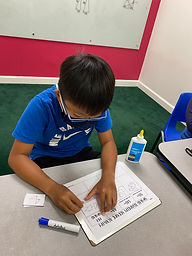## Ms. Kylie

### Target 1​

###### Lesson Type:

New

Geometry

:

Congruence

Identify similar triangles.

###### 1:

Prove that triangles are similar using SSS (Side-Side-Side), AA (Angle-Angle), and SAS (Side-Angle-Side).

###### 2:

Explain that triangles are similar if all corresponding angles are equal and all corresponding sides have the same ratio or proportion.

6th

###### Vocabulary:

Proportional sides, SSS, SAS, AA, Congruent

Activities:

-Students learned how to prove triangles are similar using SSS (Side-Side-Side), SAS (Side-Angle-Side), AA (Angle-Angle)

-Students were given different pairs of triangles to prove whether the triangles were similar and sorted them into the correct columns (SSS, SAS, AA)

-Students practiced finidng the whether the correspsonding sides to two triangles were proportional. They also learned to find corresponding congruent angles.### Home Exploration

###### Guiding Questions:## Absent Students:

### Target 2

:

###### 1:

Identify the correct arithmetic process based on the information presented in word problems.

###### 2:

Find the key words in word problems that indicate the correct arithmetic process to use.

###### 3:

Find and use the needed information in a word problem to solve.

5th

###### Vocabulary:

CUBES, Operation Words

Activities:

-Students learned to use the strategy CUBES (Circle numbers, Underline the questions, Box important words, Eliminate information, Solve) to solve multi-step word problems. Students read the problem first and then went back to use the CUBES strategy.

-Students were given word problems to solve using the strategy CUBES.### Home Exploration

###### Guiding Questions:### Target 3

:

###### Vocabulary:

Activities:### Home Exploration